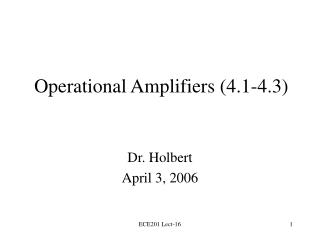DownloadDownload PresentationOperational Amplifiers (4.1-4.3)

# Operational Amplifiers (4.1-4.3)

Télécharger la présentation## Operational Amplifiers (4.1-4.3)

- - - - - - - - - - - - - - - - - - - - - - - - - - - E N D - - - - - - - - - - - - - - - - - - - - - - - - - - -
##### Presentation Transcript

1. Operational Amplifiers (4.1-4.3) Dr. Holbert April 3, 2006 ECE201 Lect-16

2. Op Amps • Op Amp is short for operational amplifier. • An operational amplifier is modeled as a voltage controlled voltage source. • An operational amplifier has a very high input impedance and a very high gain. ECE201 Lect-16

3. Use of Op Amps • Op amps can be configured in many different ways using resistors and other components. • Most configurations use feedback. ECE201 Lect-16

4. Applications of Op Amps • Amplifiers provide gains in voltage or current. • Op amps can convert current to voltage. • Op amps can provide a buffer between two circuits. • Op amps can be used to implement integrators and differentiators. • Lowpass and bandpass filters. ECE201 Lect-16

5. + - The Op Amp Symbol High Supply Non-inverting input Output Inverting input Ground Low Supply ECE201 Lect-16

6. The Op Amp Model v+ Non-inverting input + vo Rin + – Inverting input – A(v+ -v- ) v- ECE201 Lect-16

7. Typical Op Amp • The input resistance Rin is very large (practically infinite). • The voltage gain A is very large (practically infinite). ECE201 Lect-16

8. “Ideal” Op Amp • The input resistance is infinite. • The gain is infinite. • The op amp is in a negative feedback configuration. ECE201 Lect-16

9. The Basic Inverting Amplifier R2 R1 – + – + + Vin Vout – ECE201 Lect-16

10. Consequences of the Ideal • Infinite input resistance means the current into the inverting input is zero: i- = 0 • Infinite gain means the difference between v+ and v- is zero: v+ - v- = 0 ECE201 Lect-16

11. Solving the Amplifier Circuit Apply KCL at the inverting input: i1 + i2 + i-=0 R2 i2 R1 – i1 i- ECE201 Lect-16

12. KCL ECE201 Lect-16

13. Solve for vout Amplifier gain: ECE201 Lect-16

14. Recap • The ideal op-amp model leads to the following conditions: i- = 0 = i+ v+ = v- • These conditions are used, along with KCL and other analysis techniques, to solve for the output voltage in terms of the input(s). ECE201 Lect-16

15. Where is the Feedback? R2 R1 – + – + + Vin Vout – ECE201 Lect-16

16. Review • To solve an op-amp circuit, we usually apply KCL at one or both of the inputs. • We then invoke the consequences of the ideal model. • The op amp will provide whatever output voltage is necessary to make both input voltages equal. • We solve for the op-amp output voltage. ECE201 Lect-16

17. The Non-Inverting Amplifier + + – + – vin vout R2 R1 – ECE201 Lect-16

18. KCL at the Inverting Input + + – + – i- vin vout i1 i2 R2 R1 – ECE201 Lect-16

19. KCL ECE201 Lect-16

20. Solve for Vout ECE201 Lect-16

21. A Mixer Circuit R1 Rf + – R2 v1 – + – + + v2 vout – ECE201 Lect-16

22. KCL at the Inverting Input R1 Rf i1 if + – R2 v1 i2 – i- + – + + v2 vout – ECE201 Lect-16

23. KCL ECE201 Lect-16

24. KCL ECE201 Lect-16

25. Solve for Vout ECE201 Lect-16

26. Class Example • Learning Extension E4.1 • Learning Extension E4.2 • Learning Extension E4.3 ECE201 Lect-16